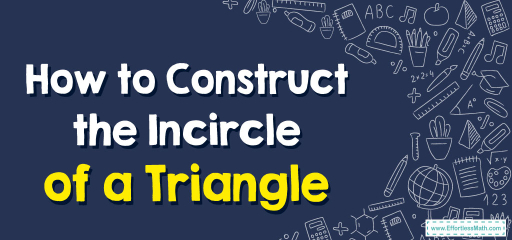# How to Construct the Incircle of a Triangle

In the intricate world of geometry, triangles hold a special place. They are more than mere shapes with three sides and three angles. One fascinating aspect of triangles is the incircle, a circle that's perfectly positioned inside, gently touching all three of its sides. While the concept of this incircle may initially appear intricate, with a clear approach and the right methods, its construction is both engaging and insightful. In this guide, we'll delve into a clear and detailed breakdown, ensuring you grasp the underlying mathematics and steps involved in crafting the incircle of a triangle.## Step-by-step Guide: The Inscribed Circle of a Triangle

Tools Required:

• Straightedge or Ruler: For creating straight lines and measurements.
• Compass: Indispensable for constructing arcs and circles.
• Pencil: For marking and drawing.

Procedure:

1. Drawing the Base: Start with a triangle $$ABC$$, which will serve as the foundation for our subsequent geometric constructions.

2. Angle Bisectors:
– For vertex $$A$$, place the compass on $$A$$ and draw an arc intersecting sides $$AB$$ and $$AC$$, creating points $$D$$ on $$AB$$ and $$E$$ on $$AC$$. Connect $$A$$ to the point where the arcs intersect, creating angle bisector $$AD$$.
– Repeat this process for vertices $$B$$ and $$C$$ to get their respective angle bisectors.

3. Identifying the Incenter:
– The incenter, denoted as $$I$$, is the intersection point of the angle bisectors.
– This point is equidistant from all three sides of the triangle.

4. Constructing the Incircle:
– Set the compass width to the distance between the incenter $$I$$ and any side of the triangle, say $$BC$$. This distance is the inradius.
– With the compass point at $$I$$, draw a circle. This circle, which touches all three sides of $$ABC$$, is the triangle’s incircle.

### Examples

Example 1:
Triangle $$DEF$$ is a right triangle at $$E$$ with sides $$DE = 3 \text{ cm}$$, $$EF = 4 \text{ cm}$$, and $$DF = 5 \text{ cm}$$.

Solution:
Draw triangle $$DEF$$ and follow the above steps to find its incenter and inradius.
The incenter will lie on the midpoint of the hypotenuse $$DF$$, and the inradius will be half the difference between the hypotenuse’s length and the sum of the other two sides.

Example 2:
Triangle $$GHI$$ is isosceles with $$GH = GI = 6 \text{ cm}$$ and $$HI = 8 \text{ cm}$$.

Solution:
After sketching triangle $$GHI$$, construct the incircle.
Due to the symmetry of the isosceles triangle, the incenter will reside on the median from the vertex angle.

### Practice Questions:

1. Can an obtuse triangle have an incircle that touches all its sides? Explain.
2. Given an equilateral triangle of side length $$9 \text{ cm}$$, how would you find its inradius without constructing the incircle?
3. Does the incenter of a triangle always lie within the triangle?

1. Yes, every triangle, including obtuse triangles, has a unique incircle that touches all its sides.
2. For an equilateral triangle with side length $$a$$, the inradius $$r$$ can be found using the formula:
$$r = \frac{a}{2\sqrt{3}}$$
For $$a = 9 \text{ cm}$$, $$r = \frac{9}{2\sqrt{3}} = \frac{9\sqrt{3}}{6} = 1.5\sqrt{3} \text{ cm}$$.
3. Yes, the incenter always lies inside the triangle.

### What people say about "How to Construct the Incircle of a Triangle - Effortless Math: We Help Students Learn to LOVE Mathematics"?

No one replied yet.

X
30% OFF

Limited time only!

Save Over 30%

SAVE $5 It was$16.99 now it is \$11.99